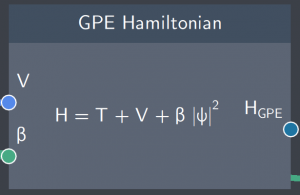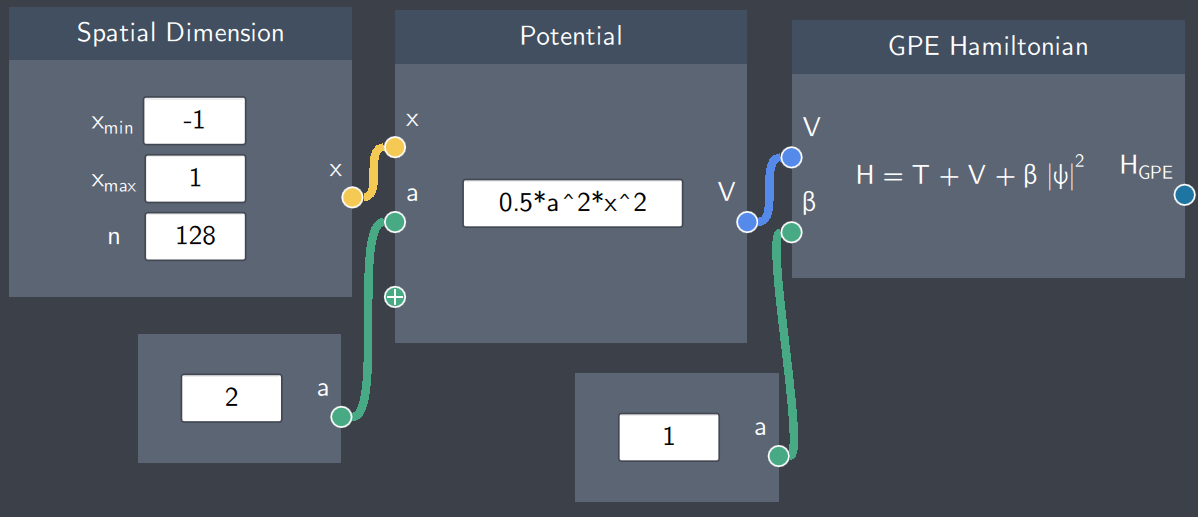# GPE Hamiltonian

## Description

The GPE Hamiltonian node defines the total energy of the system modelled by the Gross-Pitaevskii equation describing the mean field of Bose-Einstein condensates. It is the first building block to get the energy spectrum of a system.## Inputs

The node has the following inputs:

• Potental (V): A potential function defined in the Potential node
• Self-interaction ($\beta$): A scalar quantity denoting the interaction strength of the condensate

## Content

The content in the node shows what is being calculated. It is the sum of the kinetic and potential energy and a non-linear term describing the self-interaction strength.

## Output

The node outputs the Hamiltonian based on the GPE

## Example

In the example below, the GPE Hamiltonian has a harmonic oscillator potential as the potential function and the self-interaction strength of the system is equal to 1.